Courses

# SSC CPO Mock Test (GI & Reas) 1

## 50 Questions MCQ Test SSC CPO & Constable - Mock Tests & Previous Year Papers | SSC CPO Mock Test (GI & Reas) 1

Description
This mock test of SSC CPO Mock Test (GI & Reas) 1 for SSC helps you for every SSC entrance exam. This contains 50 Multiple Choice Questions for SSC SSC CPO Mock Test (GI & Reas) 1 (mcq) to study with solutions a complete question bank. The solved questions answers in this SSC CPO Mock Test (GI & Reas) 1 quiz give you a good mix of easy questions and tough questions. SSC students definitely take this SSC CPO Mock Test (GI & Reas) 1 exercise for a better result in the exam. You can find other SSC CPO Mock Test (GI & Reas) 1 extra questions, long questions & short questions for SSC on EduRev as well by searching above.
QUESTION: 1

Solution:
QUESTION: 2

Solution:
QUESTION: 3

### Find out which word or name will be in the middle after the words or names are arranged in the alphabetical order.

Solution:
QUESTION: 4
Choose the word which is least like the other words in the group .
Solution:
QUESTION: 5
Choose the word which is least like the other words in the group .
Solution:
QUESTION: 6
Choose the word which is least like the other words in the group .
Solution:
QUESTION: 7
Complete the analogous pair.
Calf : Cow : : Puppy : ?
Solution:
QUESTION: 8
Complete the analogous pair.
Deep : Shallow : : Freedom : ?
Solution:
QUESTION: 9
Complete the analogous pair.
Wine : Grapes : : Vodka : ?
Solution:
QUESTION: 10
Complete the analogous pair.
Wrist : Elbow : : Ankle : ?
Solution:
QUESTION: 11
A and B starts moving towards each other from two places 200 m apart. After walking 60 m, B turns left and goes 20 m, then he turns right and goes 40 m. He then turns right again and comes back to the road on which he had started walking. If A and B walk with the same speed, what is the distance between A and B now ?
Solution:
QUESTION: 12
Five girls are standing in a row facing East. Savita is to the left of Usha, Tulsa and Urmila. Usha, Tulsa and Urmila are to the left of Kumud. Urmila is between Usha and Tulsa. If Tulsa is fourth from the left, how far is Usha from the right?
Solution:
QUESTION: 13
In the following alphabetical series, which letter will be 8th letter from the left of 6th letter from the right side?
A B C D..........Z.
Solution:
QUESTION: 14

How many triangles are there in the following figure?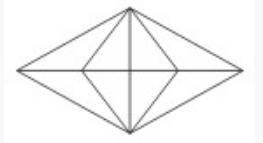Solution:

There are eight triangles in the upper half of the figure, namely - ABE, AEO, ABO, AOF, AFC, AOC, AEF and ABC

Similarly, there are eight triangles in the lower half of the figure
There are four more triangles, namely : ABD, AED, AFD and ACD
Hence there are a total of twenty triangles in the figure.

QUESTION: 15

Find the missing character.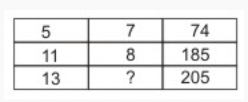Solution:
QUESTION: 16

Find the missing character.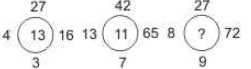Solution:

In fig-I 3 × 9 = 27
4 × 4 = 16
9 + 4 = 13
In fig-II 13 × 5 = 65
7 × 6 = 42
5 + 6 = 11
In fig-III 9 × 3 = 27
8 × 9 = 72
9 + 3 = 12

QUESTION: 17
Group the following figures into three classes on the basis of identical properties.
Solution: Image 1, 3 and 5 are circle related
Image 2, 6, 8 are triangle
Image related 4, 7, 9 are quadrilateral
QUESTION: 18

Find the missing character.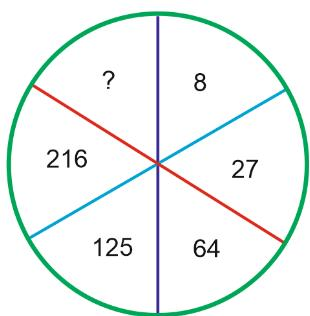Solution:

Moving clockwise, we get the squence : 23 , 33 , 43 , 53 , 63 , 73 .

QUESTION: 19

Choose the odd one out.

Solution:
QUESTION: 20

Which is the one that does not belong to the group?

Solution:
QUESTION: 21

If it is possible to make a meaningful word with the first, the fifth, the ninth and the eleventh letters of the word PENULTIMATE using each letter only one, which of the following will be the third letter of that word? If no such word can be made give 'N' as the answer and if more than one such word can be formed give 'D' as the answer.

Solution:

The letters are: P, L, A, E. Meaningful words: PALE, LEAP, PEAL.

QUESTION: 22

In a certain code language the letters immediate next to vowels are replaced with D, all other consonants with preceding letters and the vowels are replaced with Z. How will the word STANDING be written in that code language?

Solution:

Vowels → AEIOU
letters immediate to vowels → B F J P V . And these letters should be replaced with D in the given word ‘STANDING’
But there is no such letter in the given word.
Consonants in ‘STANDING’ → STNDG
Preceding letters to STNDG → RSMCF
Vowels in ‘STANDING’ → A I ( We have to write Z in the place of these vowels)
So, the new code word for ‘STANDING’ is → ‘RSZMCZMF’

QUESTION: 23

In a certain code SEAL is written as \$75@ and DOSE is written as #8\$7. How is SOLD written in that code?

Solution:
QUESTION: 24

In a certain code DEAL is written as '3524' and LIE is written as '475'. How is IDLE written in that code?

Solution:

D → 3
E → 5
A → 2
L → 4
I → 7
Hence,
I → 7
D → 3
L → 4
E → 5

QUESTION: 25

In the given diagram, Circle A represents teachers who can teach physics, Circle B represents teachers who can teach chemistry and Circle C represents those who can teach Mathematics. Among the region marked p, q, r, .....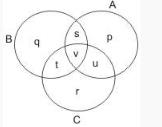The one which represents teachers who can teach physics and mathematics but not chemistry, is

Solution:

The regions common to circle A (Physics) and circle C ( Mathematics )are v and u where v represents teachers who can teach all subject and u represents the teachers who can teach Physics and Mathematics but not Chemistry.

QUESTION: 26

If + means -, - means ×, × means ÷ and ÷ means +, then
30 ÷ 6 + 2 - 1 = ?

Solution:

30 + 6 - 2 x 1
= 30 + 6 - 2
= 36 - 2 = 34

QUESTION: 27

If * means “is greater than”, @ means `is less then’; and \$ means “is equal to” and if a \$ b and b @ c, then

Solution:

Replace the symbols with the meaning given against them. If a \$ b and b @ c would become a = b and b < c, then c > b or c > a ie, c * b, c * a are true from the given options.

QUESTION: 28

If × means ÷, - means ×, ÷ means + and + means -, then
(3 - 15 ÷ 19) × 8 + 6 = ?

Solution:

Given expression : (3 × 15 + 19) ÷ 8 - 6 = (45 + 19) ÷ 8 - 6 = 64 ÷ 8 - 6 = 8 - 6 = 2

QUESTION: 29

Complete the analogous pair.
42 : 56 :: 110 : ?

Solution:

42 : 56 :: 110 : ?
6 × 7 = 42
7 × 8 = 56
Similarly,
10 × 11 = 110
11 × 12 = 132
Hence, 110 : 132 as 42 : 56

QUESTION: 30

Choose the odd one out.

Solution:
QUESTION: 31
Choose the odd one out.
Solution:
QUESTION: 32

Choose the odd one out.

Solution:

1 : 4
1 × 2 + 2 = 4
8 : 18
8 × 2 + 2 = 18
22 : 46
22 × 2 + 2 = 46
50 : 102
50 × 2 + 2 = 102
10 : 24
10 × 2 + 4 = 24

QUESTION: 33
Which one set of letters when sequentially placed at the gaps in the given letter series shall complete it?
ae-ga-eg-ce-
Solution:
QUESTION: 34
Find out from amongst the four alternatives as to how the pattern would appear when the transparent sheet is folded at the dotted line.
Solution:
QUESTION: 35
Find the wrong number in the series.
16, 19, 21, 30, 46, 71, 107
Solution: Difference between 1st no and 2nd no is 12
Difference between 2nd no and 3rd no is 22
Difference between 3rd no and 4th no is 32
and so on
So 19 is the wrong no.
Correct no is 17
QUESTION: 36
2, 3, (6), 11, 18, (30), 38
In the above number series TERMS have been put within bracket. Mark your answer as -
Solution:
QUESTION: 37
In this letter series, some of the letters are missing. Choose the correct letter given below -
_ bca _ cca _ ca _ b _ c
Solution:
QUESTION: 38
In this letter series, some of the letters are missing. Choose the correct letter given below -
aa _ ab _ _ aaa _ a
Solution:
QUESTION: 39
Below given question a statement followed by two assumptions numbered I and II. Consider the statement and decide which of the given assumption is implicit.
Statement :
Never before such a lucid book was available on the topic.
Assumptions :
I. Some other books were available on this topic.
II. You can get lucid books on very few topics.
Solution:
QUESTION: 40
Choose the correct alternative that will continue the same pattern and fill in the blank.
1, 2, 6, 24, (.....)
Solution:
QUESTION: 41
Below is given statement followed by four conclusions numbered I, II, III and IV. You have to consider the statement and the following conclusions and decide which of the conclusions is follows in the statement :
Statements : a. All lions are ducks.
b. No duck is a horse.
c. All horses are fruits.
Conclusions : I. No lion is a horse.
II. Some fruits are horses.
III. Some ducks are lions.
IV. Some lions are horses.
Solution:
QUESTION: 42

If blue means pink, pink means green, green means yellow, yellow means red and red means white then what is the colour of turmeric?

Solution:
QUESTION: 43
Romit remembers that Lokesh's birthday is after 19th but before 22nd November, where as Deepti remembers that Lokesh's birthday is after 20th but before 24th November. Which day is Lokesh's birthday?
Solution:
QUESTION: 44

In each question, five words are given. Four of them are alike in some way. One is different from them.Which is the one that does not belong to that group?

Solution:
QUESTION: 45

Select a figure from the alternatives, which when placed in the blank space of figure (X) would complete the pattern.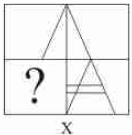Solution:
QUESTION: 46

Study the following diagram.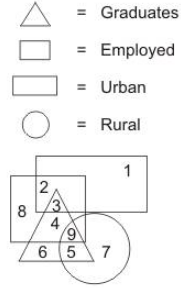Q. What does number 4 represent?

Solution:
QUESTION: 47

Select the correct mirror-image of the Figure (X) from amongst the given alternatives.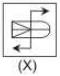Solution:
QUESTION: 48

Each of the following question consist of five problem figure marked 1, 2, 3, 4, 5 followed by Answer figure A, B, C, D and E. Select of figure from the answer figure which will continue the same series as given in the problem figure.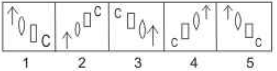Solution:
QUESTION: 49

In the questions, figure (X) is embedded in one of following figures. Find out the correct alternative.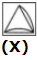Solution:
QUESTION: 50

The following questions are based on the five three letter words given below:
FAN HOP GET CUB MID
(NOTE : The words formed after performing the given operations may or may not be meaningful English words.)

Q. If the positions of the first and second letters of all the words are interchanged, how many words will form meaningful english words?

Solution: